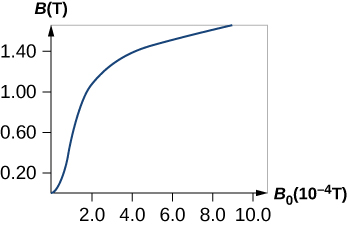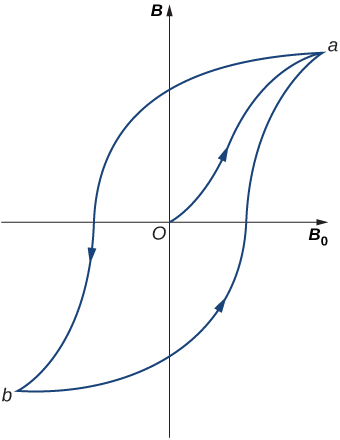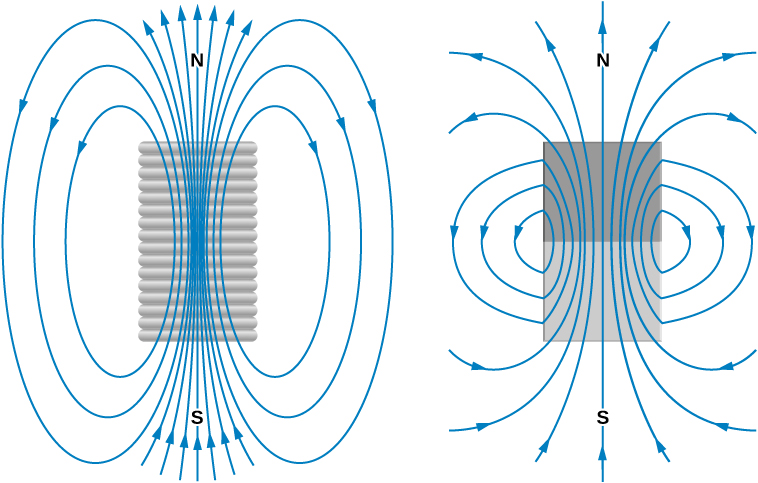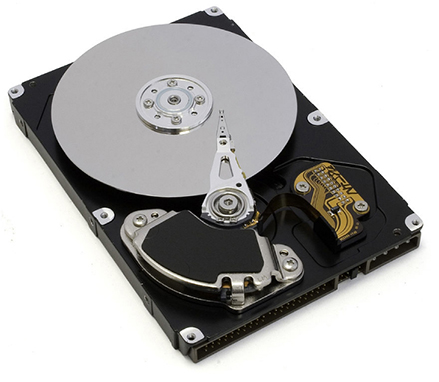# 12.7 Magnetism in matter  (Page 4/13)

 Page 4 / 13(a) The magnetic field B in annealed iron as a function of the applied field B 0 .

When ${B}_{0}$ is varied over a range of positive and negative values, B is found to behave as shown in [link] . Note that the same ${B}_{0}$ (corresponding to the same current in the solenoid) can produce different values of B in the material. The magnetic field B produced in a ferromagnetic material by an applied field ${B}_{0}$ depends on the magnetic history of the material. This effect is called hysteresis    , and the curve of [link] is called a hysteresis loop. Notice that B does not disappear when ${B}_{0}=0$ (i.e., when the current in the solenoid is turned off). The iron stays magnetized, which means that it has become a permanent magnet.A typical hysteresis loop for a ferromagnet. When the material is first magnetized, it follows a curve from 0 to a . When B 0 is reversed, it takes the path shown from a to b . If B 0 is reversed again, the material follows the curve from b to a .

Like the paramagnetic sample of [link] , the partial alignment of the domains in a ferromagnet is equivalent to a current flowing around the surface. A bar magnet can therefore be pictured as a tightly wound solenoid with a large current circulating through its coils (the surface current). You can see in [link] that this model fits quite well. The fields of the bar magnet and the finite solenoid are strikingly similar. The figure also shows how the poles of the bar magnet are identified. To form closed loops, the field lines outside the magnet leave the north (N) pole and enter the south (S) pole, whereas inside the magnet, they leave S and enter N.Comparison of the magnetic fields of a finite solenoid and a bar magnet.

Ferromagnetic materials are found in computer hard disk drives and permanent data storage devices ( [link] ). A material used in your hard disk drives is called a spin valve, which has alternating layers of ferromagnetic (aligning with the external magnetic field) and antiferromagnetic (each atom is aligned opposite to the next) metals. It was observed that a significant change in resistance was discovered based on whether an applied magnetic field was on the spin valve or not. This large change in resistance creates a quick and consistent way for recording or reading information by an applied current.The inside of a hard disk drive. The silver disk contains the information, whereas the thin stylus on top of the disk reads and writes information to the disk.

## Iron core in a coil

A long coil is tightly wound around an iron cylinder whose magnetization curve is shown in [link] . (a) If $n=20$ turns per centimeter, what is the applied field ${B}_{0}$ when ${I}_{0}=0.20\phantom{\rule{0.2em}{0ex}}\text{A}?$ (b) What is the net magnetic field for this same current? (c) What is the magnetic susceptibility in this case?

## Strategy

(a) The magnetic field of a solenoid is calculated using [link] . (b) The graph is read to determine the net magnetic field for this same current. (c) The magnetic susceptibility is calculated using [link] .

## Solution

1. The applied field ${B}_{0}$ of the coil is
$\begin{array}{ccc}\hfill {B}_{0}& =\hfill & {\mu }_{0}n{I}_{0}=\left(4\pi \phantom{\rule{0.2em}{0ex}}×\phantom{\rule{0.2em}{0ex}}{10}^{-7}\text{T}·\text{m/A}\right)\left(2000\phantom{\rule{0.1em}{0ex}}\text{/}\phantom{\rule{0.1em}{0ex}}\text{m}\right)\left(0.20\phantom{\rule{0.2em}{0ex}}\text{A}\right)\hfill \\ \hfill {B}_{0}& =\hfill & 5.0\phantom{\rule{0.2em}{0ex}}×\phantom{\rule{0.2em}{0ex}}{10}^{-4}\text{T}.\hfill \end{array}$
2. From inspection of the magnetization curve of [link] , we see that, for this value of ${B}_{0},$ $B=1.4\phantom{\rule{0.2em}{0ex}}\text{T}.$ Notice that the internal field of the aligned atoms is much larger than the externally applied field.
3. The magnetic susceptibility is calculated to be
$\chi =\frac{B}{{B}_{0}}-1=\frac{1.4\phantom{\rule{0.2em}{0ex}}\text{T}}{5.0\phantom{\rule{0.2em}{0ex}}×\phantom{\rule{0.2em}{0ex}}{10}^{-4}\text{T}}\text{−1}=2.8\phantom{\rule{0.2em}{0ex}}×\phantom{\rule{0.2em}{0ex}}{10}^{3}.$

## Significance

Ferromagnetic materials have susceptibilities in the range of ${10}^{3}$ which compares well to our results here. Paramagnetic materials have fractional susceptibilities, so their applied field of the coil is much greater than the magnetic field generated by the material.

Why does the lines of force not touch each other 🇲🇲
what is unit
Please canu get more questions on electric field and electric flux please
Gbemisola
is electric field directly proportional to the squared of a distance
No electric field is inversely proportional to the squared distance between the charges
Gbemisola
The bullet 2.00cm long is fired at 420/s and passes straight through a 10.0 cm thick board existing at 280 m/s.What is the average acceleration of the bullet through the board?
FAUSTINA
an unstretched spring is 12cm long .A load of 5N stretched it to 15cm .how long will it be under a load of 15N?
Morapeli
hi
Africa
hi
Benjamin
Benjamin how are u are u a freshman in the university
Africa
like 100 level
Africa
yes sir
Benjamin
l need a physics tutor
Benjamin
I think the best tutor is God and organic tutor in YouTube that guy is good
Africa
me too 100level
Africa
wow nice
Benjamin
from Nigeria and u
Africa
I am from Nigeria and u wow nice that something I use to always say
Africa
am from Ghana
Benjamin
ok
Africa
studying what
Africa
Compare the electric flux through the surface of a cube of side length a that has a charge q at its center to the flux through a spherical surface of radius a with a charge q at its center.
please I want to know how to solve increase in length
Ujah
Why a charged capacitor has potential difference but not emf
what is the dimension symbol of temperature?
what is the dimension symbol of temperature?
Keren
what's the meaning of enthalpy in terms of latent heat, internal energy, phase change
Enthalpy is the degree ofdisorderlinessof a substance
Gbemisola
how to convert Kelvin to centigrade
what is the s, p, d, f in this table
Sangeetha
s, p, d, f in this table
Sangeetha
what kind of table this
Vengata
Periodic table
Gbemisola
what are waves
In physics, mathematics, and related fields, a wave is a propagating dynamic disturbance (change from equilibrium) of one or more quantities
Discuss how would orient a planar surface of area A in a uniform electric field of magnitude E0 to obtain (a) the maximum flux and (b) the minimum flux through the area.
I'm just doing the first 3 with this message. but thankyou for the time your obviously intending to support us with. viva la accumulation
Marcel
Find the net capacitance of the combination of series and parallel capacitors shown belo
what is ohm?
calculate ideal gas pressure of 0.300mol,v=2L T=40°c
what is principle of superposition

#### Get Jobilize Job Search Mobile App in your pocket Now!By Mistry BhaveshBy Maureen MillerBy OpenStaxBy OpenStaxBy Michael SagBy Saylor FoundationBy OpenStaxBy OpenStaxBy OpenStaxBy Jonathan Long